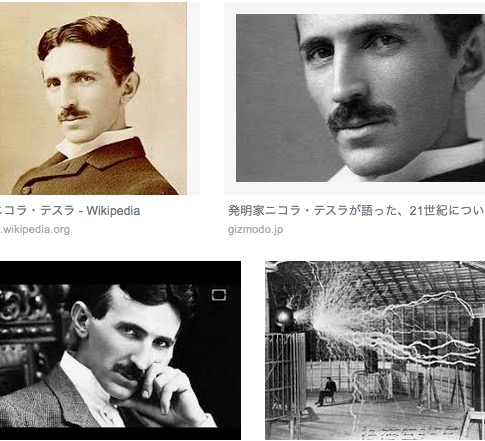# テスラの3　6　9オメガ3,6,9からの派生でテスラの3　6　9

Tesla believed that the numbers 3, 6, and 9 had extreme importance. In once quote he even stated that “If you knew the magnificence of the three, six and nine, you would have a key to the universe.” Most brushed his claims off as insanity.

What Was Nikola Tesla Trying to Say?

Math was not created by man. Instead, it was discovered. Math is the universal law of the universe, and no matter where you are, 2+2 will always equal four. This also pertains to patterns contained within the universe. Many of these patterns form the basis for sacred geometry.

One of the major patterns observed in nature is the power of binary and the power of the number two. Cells and embryos even follow this particular pattern which is: 1, 2,4,8,16,32,64,128, 256 and so on an so fourth.

According to Scientist Marko Rodin, the numbers 3, 6, and 9 compose a vector from the third to the fourth dimension which he refers to as the “flux field.” In this field, is contained a higher dimensional energy that produces the energy circuit of the following six points.

Wait, What?

For example, take the number 1. Double it, and you have 2. Double it, and you have 4. Double 4 and you get 8. Double 8 and you have 16. If you add 1+6 which are equal to 7, 16 doubled is 32 and the resulting number from 3+2 is 5. You can continue to do this and you will never reach the numbers 3, 6, and 9. No matter how far you go, none of your results will include those numbers.

However, try doubling 3. You will get six, now double 6, and you will get 12. 1+2 is 3, but in this pattern, you don’t see 9. But if you produce a pattern by use of the number 9 something else happens entirely. 9+9 is 18, 1+8 is 9. No matter how many times you do this the result will always be 9.

The Symbol of Enlightenment

If you were to go to the Pyramid of Giza, you would see that not only are there the three larger pyramids at Giza, all side by side, which mirror the positions of the stars in Orion’s Belt, but there is also a group of three smaller pyramids. If you look to nature, you will also see the use of threefold and sixfold symmetry, including the hexagonal tile shape in the honeycomb.

Could Tesla have understood something special regarding the number three and then used that understanding in his own personal scientific research?

The Magnificence of 9

To begin, think of two opposites. You can use North and South, or Light and Dark.

On one side you have 1, 2 and 4; on the other, you have 8,7, and 5. Everything in our Universe is a stream between these two sides. Similar to a pendulum, and the movement between the two would look something like the symbol of infinity.

Both sides are governed by 3 and 6. As 3 governs 1,2 and 4 while 6 governs 8, 7, and 5. If you closely enough at the pattern things become even more complicated as 1 and 2 equal 3, 2 and 4 equal 6, 4 and 8 equal 3, 8 and 7 equal 6, 7 and 5 equal 3, 5 and 1 equal 6, 1 and 2 equal 3.

The same pattern on a higher scale would be 3, 6, 3, 6, 3, 6………

And the 3 and 6 are governed by……9.

Nine would then be the unity of both sides of the equation, or the Universe itself. The vibration, the energy, and the frequency. 3, 6, and 9.

While the numbers at first seem mind boggling, they actually produce a pattern continuously that could go on forever, or times infinity. Many people may have thought Tesla to be insane, however, instead, it wasn’t that he was insane, it was that his mind thought so deeply that he had truly discovered the mathematical equation for the Universe. “The day science begins to study non-physical phenomena, it will make more progress in one decade than in all the previous centuries of its existence.”

Source: The Secret Behind the Numbers 3, 6, and 9 is Finally Revealed!

Thanks and Regards.

https://www.quora.com/Why-did-Tesla-say-that-3-6-and-9-was-the-key-to-the-universe